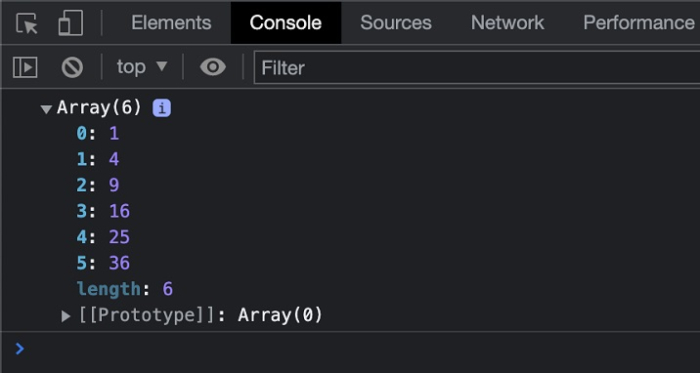# JavaScript: How to map array values without using "map" method?

We can map the elements of an array by using the looping methods in JavaScript. The map() method creates a new array that will produce the outputs of a function for each element of the array. The map method can also be implemented using the for loop in JavaScript.

## Approach

We will create two arrays out of which the first array contains the array elements that need to be mapped whereas the second array contains the output of the following array. We will be using the JavaScript Array push() function for returning the values of a function in the output array.

## Syntax

array.push(element1, element2, element, ... , elementN

For finding the length of the array, we can use the below syntax −

array.length

## Example

In the below example, we will be using the array.push() method to push the mapped values into the resultant array.

# index.html

<!DOCTYPE html>
<html>
<title>
Mapping Array Elements
</title>
<body>
<h2 style="color:green">
Welcome To Tutorials Point
</h2>
</body>
<script>
var arr = [1, 2, 3, 4, 5, 6];
var result = [];
let i;

//This function will return the square of the number
const square = function(num){
return num*num;
}
for(i=0; i< arr.length; i++){
result.push(square(arr[i]));
}
console.log(result);
//Expected output: [1, 4, 9, 16, 25, 36]
</script>
</html>

## Output